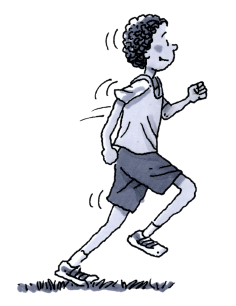### Home > ACC6 > Chapter 5 Unit 12 > Lesson CC2: 5.2.1 > Problem5-31

5-31.The Kennedy High School cross-country running team ran the following distances in recent practices:

$3.5$ miles, $2.5$ miles, $4$ miles, $3.25$ miles, $3$ miles, $4$ miles, and $6$ miles.

Find the mean and median of the team’s distances.

To find a mean, add together all of the values and divide the sum by the total number of values.